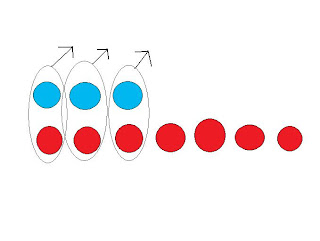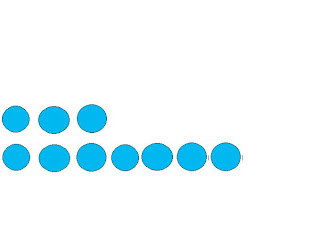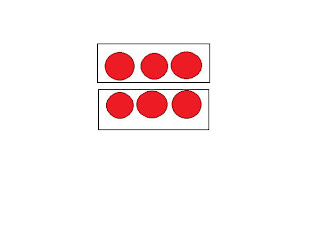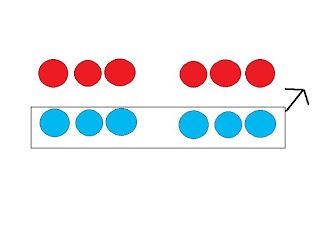## Tuesday, March 8, 2011

### Paulo's Great Big Book of Integers

Chapter 1: Gr.7 Integers Review
-Positive and Negative. When they are together it makes a zero pair.
-We can use number line for integers-"When subtracting something that isn't there, use a zero pair"

-3 - (-7) = 4-3 - 7= 103 - 7= -43 + 7= 10-3 + 7= 4

Chapter 2: Multiplying Integers The Sign Rule is something that will help you with multiplying integers.

The sign rules says when you have a even numbers of negative factors the product positive , when you have a odd number of negative factors the product is negative.

Ex.
1) (+2) x (+3)= 62) (+2) x (-3)= -63) (-2) x (+3)= 64) (-2) x (-3)= 6Chapter 3: Dividing Integers

Dividing Integers:

Partitive is figuring out how many groups there are in and how many integers are in that group.Quotative division is knowing how many integers go equally into a certain number of groups.The sign rule for division is the same as the sign rule for multiplication, addition, and subtraction.

Ex.

6 ÷ 2= 3. There is no negative sign in the question so the answer is positive.

-6 ÷ 2= 3. There is a even number of negative signs in the question, so the answer is positive.

(-6) ÷ 2= -3. There is a odd number of negative sign.

6 ÷ (-2)= -3. There is one negative sign in the question.

Chapter 4: Order of Operations with Integers

(+5) x (-3) + (-6) ÷ (+3)=
[ (+5) x (-3)] + [ (-6) ÷ (+3)]=
(-15) + (-2) = -17

Use BEDMAS.

1. Put brackets between things that needs to be multiplied and divided because you need to do the bracket numbers first.

2.Solve everything in the brackets and then rewrite everything.

3. Go through it again using BEDMAS